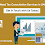## Pages

### Z-score calculation with R

Standard score or z-score is a measure of standard deviations that how much below or above the element is located from the mean value.  Z-scores are usually located around -3 to 3 sigma range (based on the variance of data, it might be different). Z-scores mean value is very close to 0, and both variance and standard deviation are equal to 1.
Z-score can be calculated with below formula,

### z = ( x - μ ) / σ

where,
x - x vector (elements of x vector)
μ - mean value of x vector
σ - standard deviation of x vector

The normal distribution curve can easily explain a z-score. Z-score values are located around the curve below. Zero is a mean center value. The highest and lowest values can be found in the right and left most parts of the curve.

Let's generate some sample data and get its z-scores.

`set.seed(123)`
`x = sample(1:50, 100, replace=T)`

Getting z-scores with a formula.

```m = mean(x)
s = sd(x)```
`zs = (x - m)/s`
```summary(x)
Min. 1st Qu.  Median    Mean 3rd Qu.    Max.
1.00   16.00   25.50   26.21   36.25   50.00```
` `
```summary(zs)
Min.  1st Qu.   Median     Mean  3rd Qu.     Max.
-1.91447 -0.77536 -0.05392  0.00000  0.76245  1.80663 ```

As summary shows, the x vector was centered into 0 mean value. In 'zs', the value of x vector's 1 is equal to -1.91, and 50 is equal to 1.8 sigma value.

In R, we can use scale() command to get z-scores.

```scale(x)
[,1]
[1,]  0.28781591
[2,] -0.69941543
[3,] -0.09188846
........
[98,]  0.51563852
[99,] -1.38288328
[100,]  0.21187503
attr(,"scaled:center")
 26.21
attr(,"scaled:scale")
 13.16814```

We need the first part of a scale function result.

```sc_zs = scale(x)[,1]
summary(sc_zs)
Min.  1st Qu.   Median     Mean  3rd Qu.     Max.
-1.91447 -0.77536 -0.05392  0.00000  0.76245  1.80663 ```

A summary shows that the result is the same as the one that was taken with a formula.
The scale function is often used to clean up data to remove the mean value from the series data.

1.2.